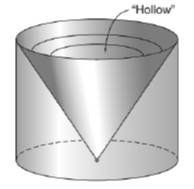Chapter 9.3, Problem 33EElementary Geometry For College St...

7th Edition
Alexander + 2 others
ISBN: 9781337614085

Solutions

Chapter
SectionElementary Geometry For College St...

7th Edition
Alexander + 2 others
ISBN: 9781337614085
Textbook Problem

A solid is formed by cutting a conical section away from a right circular cylinder. If the radius measures 6 in. and the altitude measures 8 in., what is the volume of the resulting solid?To determine

To find:

The volume of the resulting solid.

Explanation

Given:

A solid is formed by cutting a conical section away from a right circular cylinder. If the radius measures 6 in. and the altitude measures 8 in.

Formula used:

The volume of the right circular cone is V=13πr2h.

The volume of the right circular cylinder is V=πr2h.

Calculation:

Let V1 be the volume of the circular cylinder and let V2 be the volume of the right circular cone.

A solid is formed by cutting a conical section away from a right circular cylinder.

The volume of the resulting solid is V=V1V2.

We have, r=6,h=8.

First let us find V1.

V1=πr2h=π(6)28V1=288π

Now find V2

Still sussing out bartleby?

Check out a sample textbook solution.

See a sample solution

The Solution to Your Study Problems

Bartleby provides explanations to thousands of textbook problems written by our experts, many with advanced degrees!

Get Started

Polynomial Inequalities Solve the inequality. 12. 4x4 25x2 + 36 0

Precalculus: Mathematics for Calculus (Standalone Book)

In Exercise 11-14, factor the expression. y27y+494

Calculus: An Applied Approach (MindTap Course List)

Let f(x) = 1x+1 and g(x) = x2 + 1. Find the rules for (a) f + g, (b) fg (c) f t, and (d) g f.

Applied Calculus for the Managerial, Life, and Social Sciences: A Brief Approach

Simplify: 66

Elementary Technical Mathematics

Find the length of the curve y=1xt31dt1x4

Single Variable Calculus: Early Transcendentals